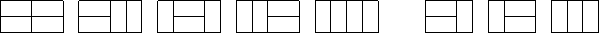[登录 · 注册]
• [POJ2411]Mondriaan's Dream
• [POJ2411]Mondriaan's Dream

试题描述

Squares and rectangles fascinated the famous Dutch painter Piet Mondriaan. One night, after producing the drawings in his 'toilet series' (where he had to use his toilet paper to draw on, for all of his paper was filled with squares and rectangles), he dreamt of filling a large rectangle with small rectangles of width 2 and height 1 in varying ways.Expert as he was in this material, he saw at a glance that he'll need a computer to calculate the number of ways to fill the large rectangle whose dimensions were integer values, as well. Help him, so that his dream won't turn into a nightmare!

输入

The input contains several test cases. Each test case is made up of two integer numbers: the height h and the width w of the large rectangle. Input is terminated by h=w=0. Otherwise, 1<=h,w<=11.

输出

For each test case, output the number of different ways the given rectangle can be filled with small rectangles of size 2 times 1. Assume the given large rectangle is oriented, i.e. count symmetrical tilings multiple times.

输入示例

```1 2
1 3
1 4
2 2
2 3
2 4
2 11
4 11
0 0```

输出示例

```1
0
1
2
3
5
144
51205```

数据规模及约定

见“输入

题解

经典的轮廓线 dp 问题。将最靠下面并且考虑过的格子的状态进行状压，即，并不是对“最后一行的状态”进行状压，而是对一个“最靠下的轮廓线”进行状压。

这里的“轮廓线”形如“上一行的 k 个元素和这一行的 m-k 个元素”。f(i, j, S) 表示考虑以 (i, j) 为右下角，轮廓线状态为 S 的方案数。具体转移参见《算法竞赛入门经典：训练指南》第六章，开头就是。

```#include <iostream>
#include <cstdio>
#include <cstdlib>
#include <cstring>
#include <cctype>
#include <algorithm>
using namespace std;

int x = 0, f = 1; char c = getchar();
while(!isdigit(c)){ if(c == '-') f = -1; c = getchar(); }
while(isdigit(c)){ x = x * 10 + c - '0'; c = getchar(); }
return x * f;
}

#define maxn 15
#define maxs 2048
#define LL long long

int n, m;
LL f[maxn][maxn][maxs];

int main() {
while(1) {
if(!n && !m) break;

memset(f, 0, sizeof(f));
int all = (1 << m) - 1;
f[all] = 1;
for(int i = 1; i <= n; i++)
for(int j = 1; j <= m; j++)
for(int S = 0; S <= all; S++) if(f[i][j][S]) {
if(S & 1) {
if(j < m) f[i][j+1][S>>1] += f[i][j][S];
else f[i+1][S>>1] += f[i][j][S];
}
if(!(S & 1)) {
if(j < m) f[i][j+1][S>>1|(1<<m-1)] += f[i][j][S];
else f[i+1][S>>1|(1<<m-1)] += f[i][j][S];
}
if(j > 1 && !(S >> m - 1 & 1) && (S & 1)) {
if(j < m) f[i][j+1][S>>1|(1<<m-1)|(1<<m-2)] += f[i][j][S];
else f[i+1][S>>1|(1<<m-1)|(1<<m-2)] += f[i][j][S];
}
}

printf("%lld
", f[n+1][all]);
}

return 0;
}
```
• 【推广】 阿里云小站-上云优惠聚集地（新老客户同享）更有每天限时秒杀！
【推广】 云服务器低至0.95折 1核2G ECS云服务器8.1元/月
【推广】 阿里云老用户升级四重礼遇享6.5折限时折扣！
• 原文：https://www.cnblogs.com/xiao-ju-ruo-xjr/p/6946928.html